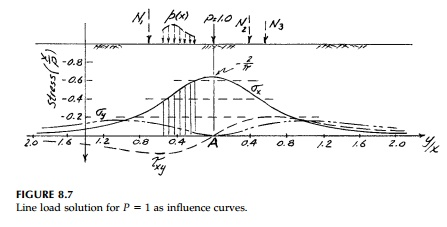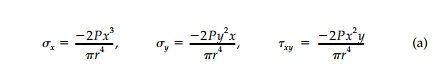Home | | Mechanics of Solids | Non uniform Loadings on the Half-Space

## Chapter: Civil : Principles of Solid Mechanics : Wedges and the Half-SpaceIt has been mentioned that this result for a uniform load on a finite width of the half-space could as easily be obtained by integrating the point-load solu-tion. This is most easily seen in terms of influence curves.

It has been mentioned that this result for a uniform load on a finite width of the half-space could as easily be obtained by integrating the point-load solu-tion. This is most easily seen in terms of influence curves. Transforming theFlamant solution [Equation (8.3)] for a normal line load to Cartesian coordi-nates by Mohr’s Circle:These components for a unit load, P=1, as plotted in Figure 8.7 are influ-ence curves in that, by reciprocity, the stress at point A (x0, 0) due to a load of magnitude N at point P (0, yo) equals, at any depth, N times the stress at point B (x0y0) due to P=1 at point 0 (0,0).

Suppose we want the stress at the depth of point A due to three normal forces N1, N2, and N3 on the surface as shown in Figure 8.7. The influence curve is located so the origin is directly above point A. The contribution to each stress component at A is then the magnitude of Ni times the ordinate of the corresponding influence curve under Ni. Therefore, to illustrate, σy at A would equal �'Ni ii or approximately N1(.3) N2(.47) N3(.35).

Similarly, by superposition, the stresses under any nonuniform normal loading can be found to any degree of accuracy desired by numerical inte-gration. The load p(x) in Figure 8.7 is simply divided into increments, x, each of which is treated as a concentrated load pLxi to be multiplied by the average ordinate of the influence curve under it. If p(x) were constant as in the previous section, then σy would equal p times the shaded area or approximately 0.5 pb.

Closed-form analytic solutions are available for a number of nonuniform strip loadings (e.g., linear, parabolic, etc.). From an engineering standpoint however, computation of stress from influence lines given by point load solutions is perfectly adequate for design and inherently more versatile. In fact, such influence lines, often called Green’s Functions, are the fundamental building blocks of the boundary element method for numerical analysis.

Another approach for nonconstant loading is to use either a Fourier or polynomial series solution. Similarly, the solutions for constant loading can be superimposed as step functions to approximate any nonconstant distribu-tion. Some of these solutions for nonuniform loading of the half-space are referred to in the chapter problems as are the influence curves for tangential loading.

Study Material, Lecturing Notes, Assignment, Reference, Wiki description explanation, brief detail
Civil : Principles of Solid Mechanics : Wedges and the Half-Space : Non uniform Loadings on the Half-Space |

Related Topics